StatLect

# Hetroskedasticity-robust standard errors

In linear regression analysis, an estimator of the asymptotic covariance matrix of the OLS estimator is said to be heteroskedasticity-robust if it converges asymptotically to the true value even when the variance of the errors of the regression is not constant.

In this case, also the standard errors, which are equal to the square roots of the diagonal entries of the covariance matrix, are said to be heteroskedasticity-robust.## The linear regression

Consider the linear regression modelwhere:

•is the dependent variable;

•is thevector of regressors;

•is thevector of regression coefficients;

•is the zero-mean error term.

## Sample

There areobservations in the sample:## The OLS estimator

The ordinary least squares (OLS) estimator ofcan be written as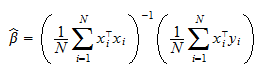## Asymptotic covariance matrix

Under appropriate conditions, the OLS estimator is asymptotically normal:where:

•denotes convergence in distribution;

•is the asymptotic covariance matrix of the OLS estimator;

•denotes a multivariate normal distribution with mean vector equal toand covariance matrix equal to.

## Standard errors

The standard errors are the estimates of the standard deviations of the entries of.

Denote byan estimator of.

Then, the covariance matrix ofis approximated byand the standard errors are equal to the square roots of the diagonal entries of the latter matrix.

## Heteroskedasticity

The errors of the regression are said to be conditionally homoskedastic if their variance is constant:whereis a constant.

If the conditional variance is not constant, the errors are said to be conditionally heteroskedastic, and the regression is said to be affected by heteroskedasticity.

## Robustness to heteroskedasticity

An estimatorof the asymptotic covariance matrixis heteroskedasticity-robust if it is consistent even when the errors are conditionally heteroskedastic.

Consistent means thatwheredenotes convergence in probability.

## Formula for the asymptotic covariance

Under mild technical conditions, the asymptotic covariance matrix iswhere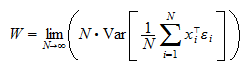is the so-called long-run covariance matrix.

## Consistent estimators

The matrixis consistently estimated byTherefore, by the Continuous Mapping Theorem, if we can find a consistent estimatorof, then the asymptotic covariance matrix is consistently estimated by## A simpler formula for the long-run covariance

Suppose that the following assumptions about the sequencehold:

1. zero mean:2. no serial correlation:if;

3. weak stationarity:does not depend onThen, the long-run covariance matrix can be written asProof

The proof is as follows: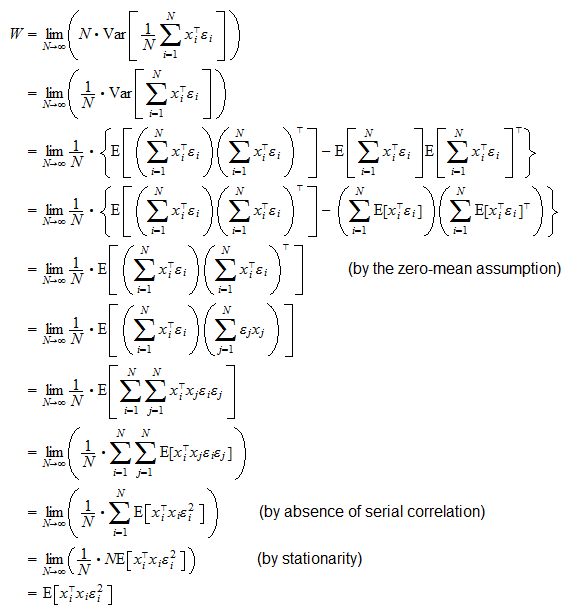Note that the zero-mean assumption is the same as the orthogonality assumption usually needed to prove the consistency of the OLS estimator.

## Estimator of the long-run covariance

Under mild technical conditions, the long-run covariance matrix is consistently estimated bywhere the residualsare defined asProof

The sample averageconverges in probability toif the sequencesatisfies the conditions of a Law of Large Numbers (the mild technical conditions mentioned above). The errorsin the last formula can be replaced by the residuals, as the latter converge in probability to the former when the sample size increases (a formal proof can be found here).

## The robust estimator

If we plug the formula forin the expression we had previously derived for the estimator of the asymptotic covariance matrix, we obtain: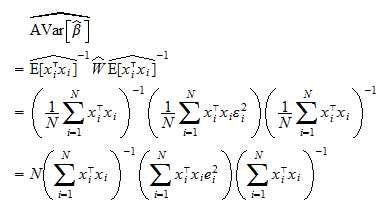This estimator is robust to heteroskedasticity.

As a matter of fact, we did not assume homoskedasticity to prove its consistency.

The square roots of the diagonal entries of the matrix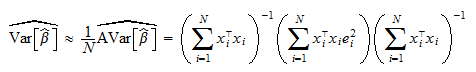are known as heteroskedasticity-robust standard errors.

## Matrix form

Using matrix notation, we can write the expression above in a more compact form.

Define the vectors and matrices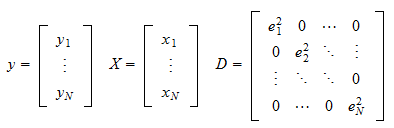Then, the heteroskedasticity-robust covariance matrix is## Non-robust estimator

Compare the formulae above with those for the non-robust estimator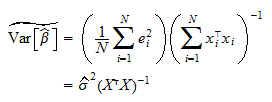whereThis estimator is non-robust to heteroskedasticity.

In fact, in order to prove its consistency, we need to assume conditional homoskedasticityfor everywithconstant.

Proof

Under the hypothesis of homoskedasticity, we can write the long-run covariance matrix as follows:which is consistently estimated byThe estimator of the asymptotic covariance matrix becomes: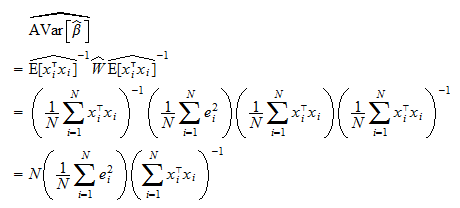Hence, the estimator of the covariance matrix is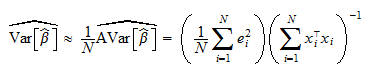## Synonyms

Heteroskedasticity-robust standard errors go by many different names:

1. heteroskedasticity-consistent standard errors;

2. Eicker-Huber-White standard errors;

3. Huber-White standard errors;

4. White standard errors.

## More details

More mathematical details and proofs of the facts stated above can be found in the lecture on the properties of the OLS estimator.

Previous entry: Realization of a random variable

Next entry: Sample mean